# 一、环境和配置

CUDA：10.1
CUDNN：7.4
python：3.7
opencv-python>=4.1.2
pytorch>=1.7.0

## 1.1 安装anaconda

anaconda的安装教程之前已经写过一篇文章，还没安装的请自行参考安装 ，这里不再赘述，参考链接：anaconda安装教程

## 1.4 安装pytorch GPU版

yolov5最新版本需要pytorch1.7（以前要求是1.6）版本以上，因此我们安装pytorch1.7版本。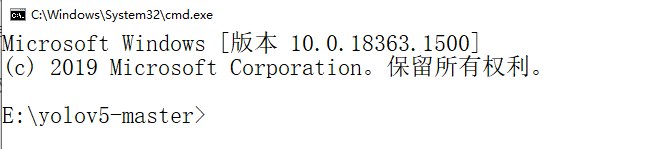``````activate torch
``````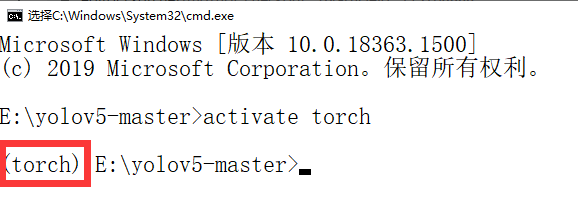``````pip install torch==1.7.0+cu101 torchvision==0.8.1+cu101 torchaudio===0.7.0 -f https://download.pytorch.org/whl/torch_stable.html
``````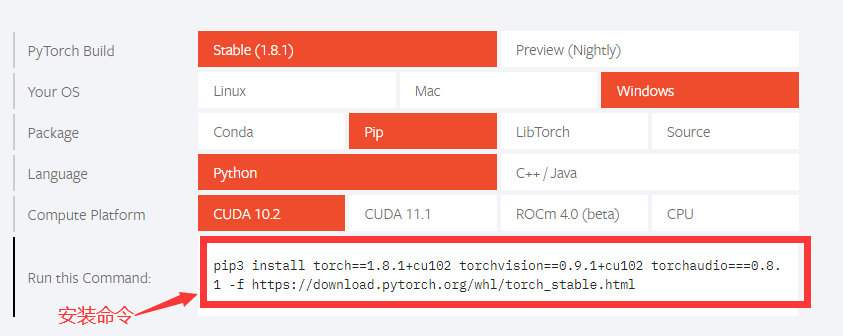``````pip install --upgrade numpy
``````

``````python
``````

``````import torch as t
t.__version__
``````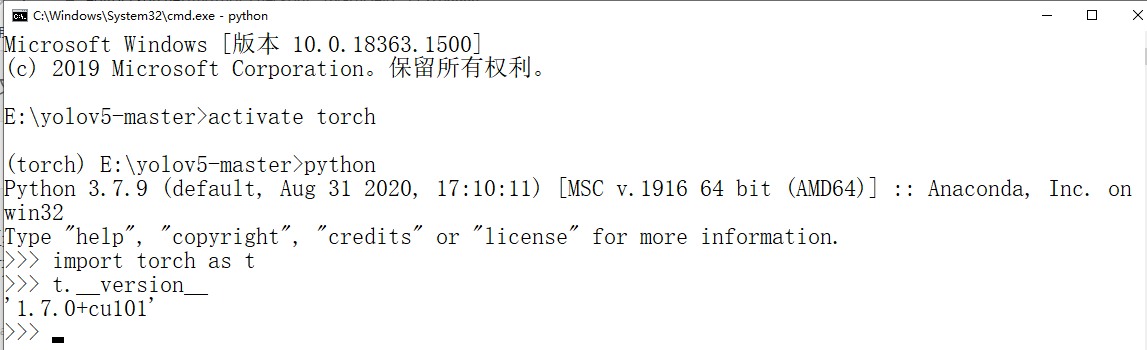``````import torch as t
t.cuda.is_available
``````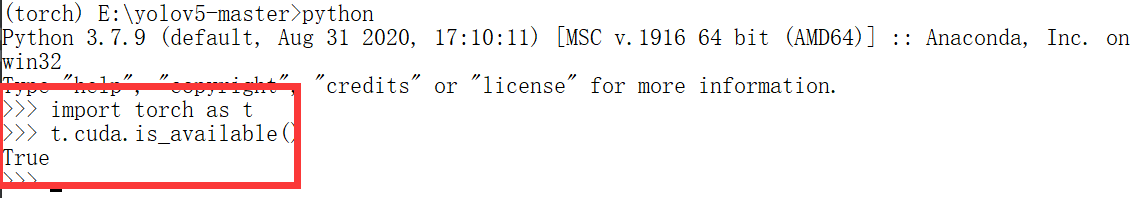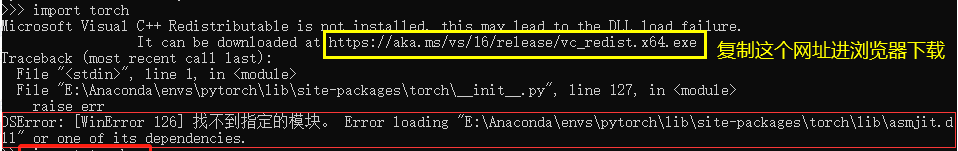# 二 源码测试

## 2.2 安装依赖项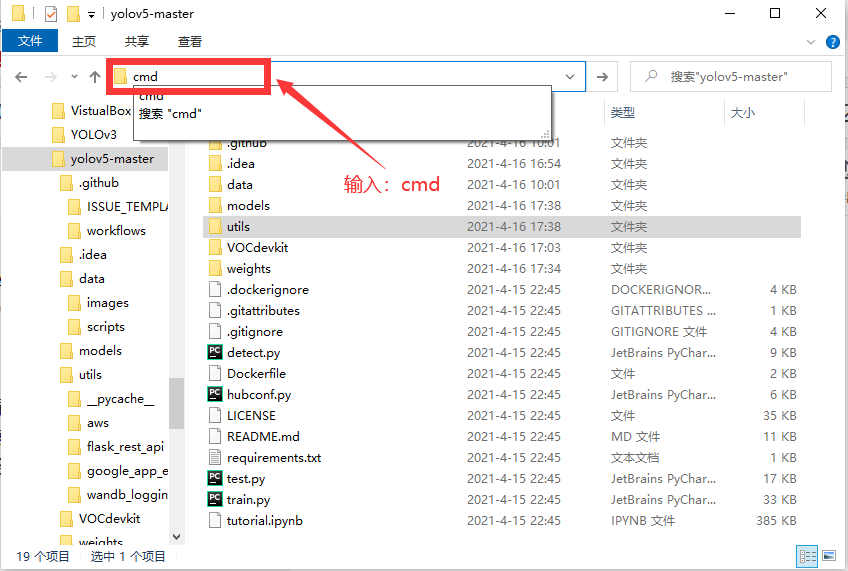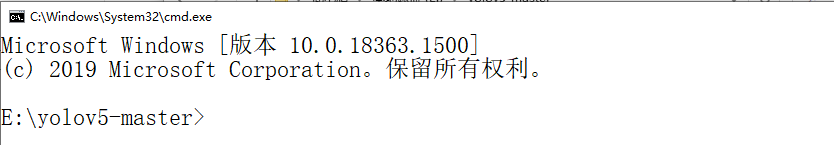``````pip install -r requirements.txt
``````

## 2.3 测试

### 2.3.1 下载权重文件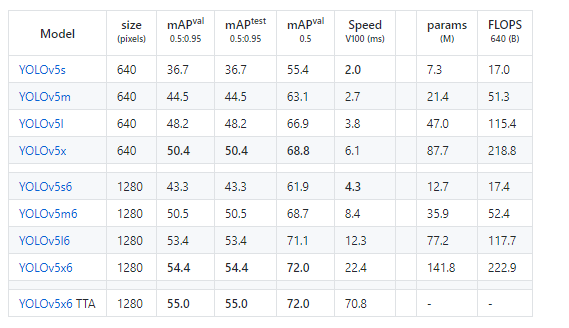### 2.3.2 测试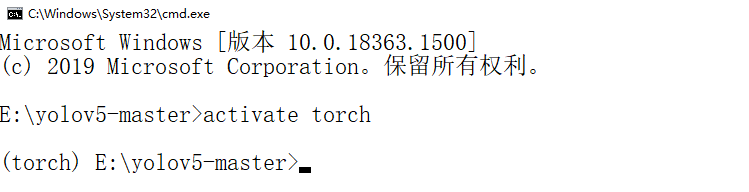``````python detect.py --source ./data/images/ --weights ./weights/yolov5s.pt --conf 0.4
``````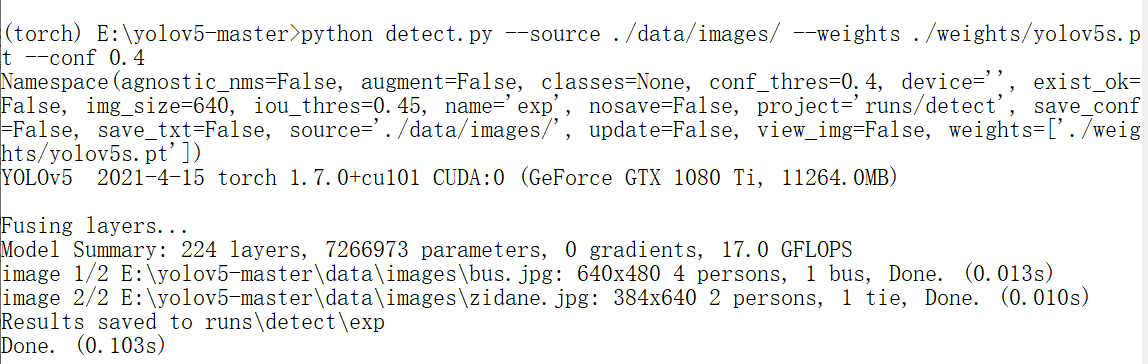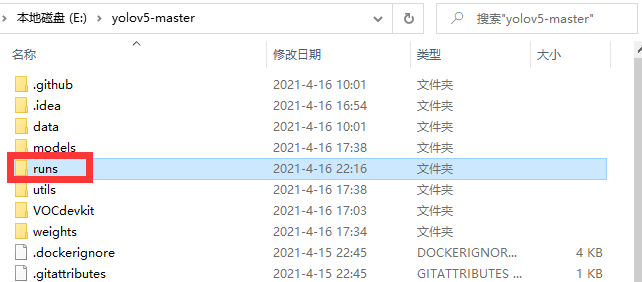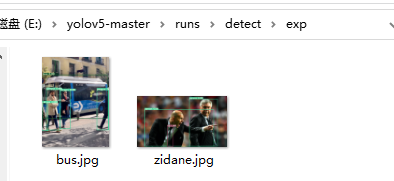``````python detect.py --source 0 --weights ./weights/yolov5s.pt --conf 0.4
``````

# 三、训练自己的数据集

## 3.1 数据集制作``````import xml.etree.ElementTree as ET
import pickle
import os
from os import listdir, getcwd
from os.path import join

sets=[('TrainVal', 'train'), ('TrainVal', 'val'), ('Test', 'test')]   # 根据自己文件夹的路径结构进行修改

classes = ['类别1', '类别2', '类别3', '类别4']  #修改为自己的类别
def convert(size, box):
dw = 1./size
dh = 1./size
x = (box + box)/2.0
y = (box + box)/2.0
w = box - box
h = box - box
x = x*dw
w = w*dw
y = y*dh
h = h*dh
return (x,y,w,h)

def convert_annotation(year, image_id):
in_file = open('VOC%s/Annotations/%s.xml'%(year, image_id))
out_file = open('VOC%s/labels/%s.txt'%(year, image_id), 'w')
tree=ET.parse(in_file)
root = tree.getroot()
size = root.find('size')
w = int(size.find('width').text)
h = int(size.find('height').text)

for obj in root.iter('object'):
difficult = obj.find('difficult').text
cls = obj.find('name').text
if cls not in classes or int(difficult) == 1:
continue
cls_id = classes.index(cls)
xmlbox = obj.find('bndbox')
b = (float(xmlbox.find('xmin').text), float(xmlbox.find('xmax').text), float(xmlbox.find('ymin').text), float(xmlbox.find('ymax').text))
bb = convert((w,h), b)
out_file.write(str(cls_id) + " " + " ".join([str(a) for a in bb]) + '\n')

wd = getcwd()

for year, image_set in sets:
if not os.path.exists('VOC%s/labels/'%(year)):
os.makedirs('VOC%s/labels/'%(year))
list_file = open('%s_%s.txt'%(year, image_set), 'w')
for image_id in image_ids:
list_file.write('%s/VOC%s/JPEGImages/%s.jpg\n'%(wd, year, image_id))
convert_annotation(year, image_id)
list_file.close()
``````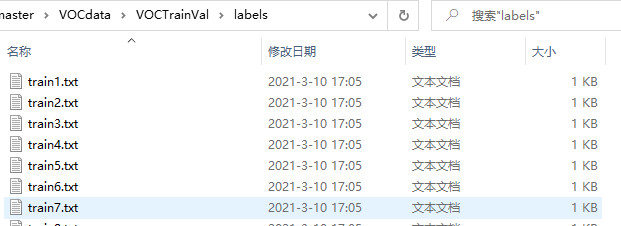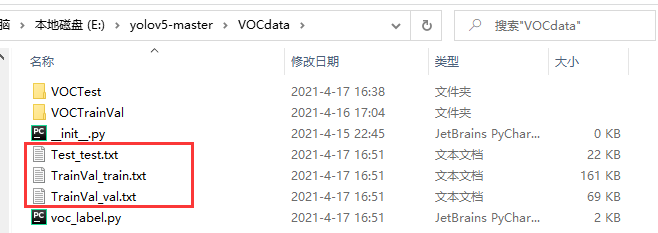## 3.2 修改配置文件

### 3.2.1 修改数据集方面的yaml文件

``````# 上面那三个文件的位置
train: ./VOCdata/TrainVal_train.txt
val: ./VOCdata/TrainVal_val.txt
test: ./VOCdata/Test_test.txt
# number of classes
nc: 4   # 修改为自己的类别数量
# class names
names: ["类别1", "类别2", "类别3", "类别4"]   # 自己来的类别名称
``````

### 3.2.2 修改网络参数方面的yaml文件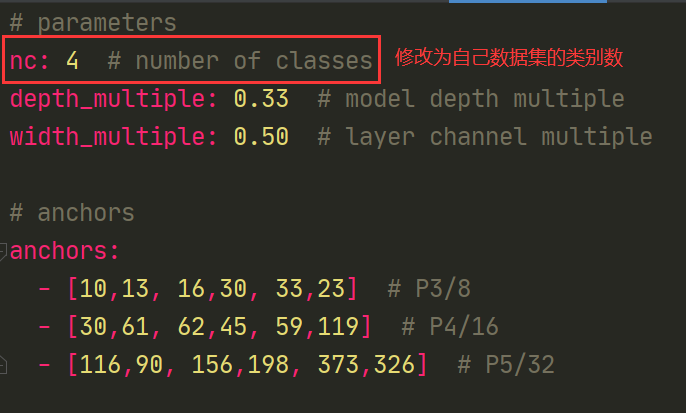### 3.2.3 修改train.py中的一些参数

``````parser.add_argument('--epochs', type=int, default=200)  # 根据需要自行调节训练的epoch
parser.add_argument('--batch-size', type=int, default=16)  # 根据自己的显卡调节，显卡不好的话	，就调小点
parser.add_argument('--cfg', type=str, default='models/yolov5s.yaml', help='*.cfg path') # 根据需要，自行选择模型
parser.add_argument('--data', type=str, default='data/myvoc.yaml', help='*.data path')  # data设置为前两步中我们新建的myvoc.yaml
parser.add_argument('--img-size', nargs='+', type=int, default=[640, 640], help='train,test sizes')  # 可调可不调
``````

## 3.3 训练

### 3.3.1 在预训练模型的基础上训练

``````python train.py --img 640 --batch 4 --epoch 300 --data ./data/myvoc.yaml --cfg ./models/yolov5s.yaml --weights weights/yolov5s.pt --workers 0
``````

–img：输入图片尺寸
–batch：batch_size大小
–epoch：训练周期
–data：上面修改过的数据集方面的信息文件
–cfg：模型的配置文件，自行选择模型，当然这里选的是哪个模型 ，就要在3.2.2中修改相应的配置文件中的类别数
–weights：预训练模型，之前下载好的，这里选择的预训练模型需要跟–cfg中相同
–workers：暂时还没搞明白是什么

### 3.3.2 从头开始训练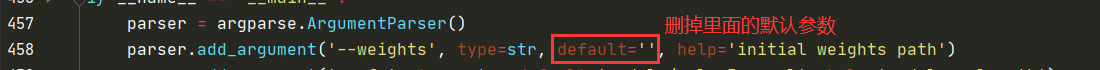``````python train.py --img 640 --batch 4 --epoch 300 --data ./data/myvoc.yaml --cfg ./models/yolov5s.yaml --workers 0
``````